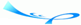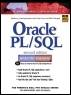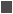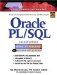# Lab 8.4 Nested Loops

 Team-FlyOracle® PL/SQL® Interactive Workbook, Second EditionBy Benjamin Rosenzweig, Elena Silvestrova Table of Contents Chapter 8.  Iterative Control

Lab Objectives

After this Lab, you will be able to:

• Use Nested Loops

You have explored three types of loops: simple loops, WHILE loops, and numeric FOR loops. Any of these three types of loops can be nested inside one another. For example, a simple loop can be nested inside a WHILE loop and vice versa. Consider the following example:FOR EXAMPLE

` DECLARE     v_counter1 INTEGER := 0;     v_counter2 INTEGER;  BEGIN     WHILE v_counter1 < 3 LOOP        DBMS_OUTPUT.PUT_LINE ('v_counter1: '||v_counter1);        v_counter2 := 0;        LOOP           DBMS_OUTPUT.PUT_LINE ('v_counter2: '||v_counter2);           v_counter2 := v_counter2 + 1;           EXIT WHEN v_counter2 >= 2;        END LOOP;        v_counter1 := v_counter1 + 1;     END LOOP;  END; `

In this example, the WHILE loop is called an outer loop because it encompasses the simple loop. The simple loop is called an inner loop because it is enclosed by the body of the WHILE loop.

The outer loop is controlled by the loop counter, v_counter1, and it will execute providing the value of v_counter1 is less than 3. With each iteration of the loop, the value of v_counter1 is displayed on the screen. Next, the value of v_counter2 is initialized to 0. It is important to note that v_counter2 is not initialized at the time of the declaration. The simple loop is placed inside the body of the WHILE loop, and the value of v_counter2 must be initialized every time before control is passed to the simple loop.

Once control is passed to the inner loop, the value of v_counter2 is displayed on the screen and incremented by 1. Next, the EXIT WHEN condition is evaluated. If the EXIT WHEN condition evaluates to FALSE, control is passed back to the top of the simple loop. If the EXIT WHEN condition evaluates to TRUE, control is passed to the first executable statement outside of the loop. In our case, control is passed back to the outer loop, and the value of v_counter1 is incremented by 1, and the test condition of the WHILE loop is evaluated again.

This logic is demonstrated by the output produced by the example:

` v_counter1: 0  v_counter2: 0  v_counter2: 1  v_counter1: 1  v_counter2: 0  v_counter2: 1  v_counter1: 2  v_counter2: 0  v_counter2: 1  PL/SQL procedure successfully completed. `

Notice that for each value of v_counter1, there are two values of v_counter2 displayed. For the first iteration of the outer loop, the value of v_counter1 is equal to 0. Once control is passed to the inner loop, the value of v_counter2 is displayed on the screen twice, and so forth.

#### Loop Labels

Earlier in the book, you read about labeling of PL/SQL blocks. Loops can be labeled in a similar manner, as follows:

` <<label_name>>  FOR LOOP_COUNTER IN LOWER_LIMIT..UPPER_LIMIT LOOP     STATEMENT 1;     …     STATEMENT N;  END LOOP label_name; `

The label must appear right before the beginning of the loop. This syntax example shows that the label can be optionally used at the end of the loop statement. It is very helpful to label nested loops because labels improve readability. Consider the following example.FOR EXAMPLE

` BEGIN     <<outer_loop>>     FOR i IN 1..3 LOOP        DBMS_OUTPUT.PUT_LINE ('i = '||i);        <<inner_loop>>        FOR j IN 1..2 LOOP           DBMS_OUTPUT.PUT_LINE ('j = '||j);        END LOOP inner_loop;     END LOOP outer_loop;  END; `

For both outer and inner loops, the statement END LOOP must be used. If the loop label is added to each END LOOP statement, it becomes easier to understand which loop is being terminated.

Loop labels can also be used when referencing loop counters.FOR EXAMPLE

` BEGIN     <<outer>>     FOR v_counter IN 1..3 LOOP        <<inner>>        FOR v_counter IN 1..2 LOOP           DBMS_OUTPUT.PUT_LINE ('outer.v_counter '||              outer.v_counter);           DBMS_OUTPUT.PUT_LINE ('inner.v_counter '||              inner.v_counter);        END LOOP inner;     END LOOP outer;  END; `

In this example, both the inner and outer loops use the same loop counter, v_counter. In order to reference both the outer and inner values of v_counter, loop labels are used. This example produces the following output:

` outer.v_counter 1  inner.v_counter 1  outer.v_counter 1  inner.v_counter 2  outer.v_counter 2  inner.v_counter 1  outer.v_counter 2  inner.v_counter 2  outer.v_counter 3  inner.v_counter 1  outer.v_counter 3  inner.v_counter 2  PL/SQL procedure successfully completed. `

Your program is able to differentiate between two variables having the same name because loop labels are used when the variables are referenced. If no loop labels are used when v_counter is referenced, the output produced by this script will change significantly. Basically, once control is passed to the inner loop, the value of v_counter from the outer loop is unavailable. When control is passed back to the outer loop, the value of v_counter becomes available again.

In this example, the same name for two different loop counters is used to demonstrate another use of loop labels. However, it is not considered a good programming practice to use the same name for different variables.

#### Lab 8.4 Exercises

##### 8.4.1 Use Nested Loops

In this exercise, you will use nested numeric FOR loops.

Create the following PL/SQL script:

` -- ch08_6a.sql, version 1.0  SET SERVEROUTPUT ON  DECLARE     v_test NUMBER := 0;  BEGIN     <<outer_loop>>     FOR i IN 1..3 LOOP        DBMS_OUTPUT.PUT_LINE('Outer Loop');        DBMS_OUTPUT.PUT_LINE('i = '||i);        DBMS_OUTPUT.PUT_LINE('v_test = '||v_test);        v_test := v_test + 1;        <<inner_loop>>        FOR j IN 1..2 LOOP           DBMS_OUTPUT.PUT_LINE('Inner Loop');           DBMS_OUTPUT.PUT_LINE('j = '||j);           DBMS_OUTPUT.PUT_LINE('i = '||i);           DBMS_OUTPUT.PUT_LINE('v_test = '||v_test);        END LOOP inner_loop;     END LOOP outer_loop;  END; `

Execute the script, and then answer the following questions:

 a) What output was printed on the screen? b) How many times was the outer loop executed? c) How many times was the inner loop executed? d) What are the values of the loop counters, i and j, after both loops terminate? e) Rewrite this script using the REVERSE option for both loops. How many times will each loop be executed in this case?

#### Lab 8.4 Exercise Answers

This section gives you some suggested answers to the questions in Lab 8.4, with discussion related to how those answers resulted. The most important thing to realize is whether your answer works. You should figure out the implications of the answers here and what the effects are from any different answers you may come up with.

##### 8.4.1 Answers

 a) What output was printed on the screen? A1: Answer: Your output should look like the following:` Outer Loop i = 1 v_test = 0 Inner Loop j = 1 i = 1 v_test = 1 Inner Loop j = 2 i = 1 v_test = 1 Outer Loop i = 2 v_test = 1 Inner Loop j = 1 i = 2 v_test = 2 Inner Loop j = 2 i = 2 v_test = 2 Outer Loop i = 3 v_test = 2 Inner Loop j = 1 i = 3 v_test = 3 Inner Loop j = 2 i = 3 v_test = 3 PL/SQL procedure successfully completed. `Every time the outer loop is run, the value of the loop counter is incremented by 1 implicitly and displayed on the screen. In addition, the value of v_test is displayed on the screen and is incremented by 1, as well. Next, control is passed to the inner loop.Every time the inner loop is run, the value of the inner loop counter is incremented by 1 and displayed on the screen, along with the value of the outer loop counter and the variable v_test. b) How many times was the outer loop executed? A2: Answer: The outer loop was executed three times, according to the range specified by the lower limit and the upper limit of the loop. In this example, the lower limit is equal to 1, and the upper limit is equal to 3. c) How many times was the inner loop executed? A3: Answer: The inner loop was executed six times.For each iteration of the outer loop, the inner loop was executed twice. However, the outer loop was executed three times. Overall, the inner loop was executed six times. d) What are the values of the loop counters, i and j, after both loops terminate? A4: Answer: After both loops terminate, both loop counters are undefined again and can hold no values. As mentioned earlier, the loop counter ceases to exist once the numeric FOR loop is terminated. e) Rewrite this script using the REVERSE option for both loops. How many times will each loop be executed in this case? A5: Answer: Your script should be similar to the script below. Changes are shown in bold letters.The outer loop will execute three times, and the inner loop will execute six times.` -- ch08_6b.sql, version 2.0 SET SERVEROUTPUT ON DECLARE v_test NUMBER := 0; BEGIN <> FOR i IN REVERSE 1..3 LOOP DBMS_OUTPUT.PUT_LINE('Outer Loop'); DBMS_OUTPUT.PUT_LINE('i = '||i); DBMS_OUTPUT.PUT_LINE('v_test = '||v_test); v_test := v_test + 1; <> FOR j IN REVERSE 1..2 LOOP DBMS_OUTPUT.PUT_LINE('Inner Loop'); DBMS_OUTPUT.PUT_LINE('j = '||j); DBMS_OUTPUT.PUT_LINE('i = '||i); DBMS_OUTPUT.PUT_LINE('v_test = '||v_test); END LOOP inner_loop; END LOOP outer_loop; END; `This script produces the following output:` Outer Loop i = 3 v_test = 0 Inner Loop j = 2 i = 3 v_test = 1 Inner Loop j = 1 i = 3 v_test = 1 Outer Loop i = 2 v_test = 1 Inner Loop j = 2 i = 2 v_test = 2 Inner Loop j = 1 i = 2 v_test = 2 Outer Loop i = 1 v_test = 2 Inner Loop j = 2 i = 1 v_test = 3 Inner Loop j = 1 i = 1 v_test = 3 PL/SQL procedure successfully completed. `Notice that the output produced by this example has changed significantly from the output in the previous example. The values of the loop counters are decremented because the REVERSE option is used. However, the value of the variable v_test was not affected by using the REVERSE option.

#### Lab 8.4 Self-Review Questions

In order to test your progress, you should be able to answer the following questions.

Answers appear in Appendix A, Section 8.4.

 1) What types of PL/SQL loop can be nested one inside another? _____ A simple loop can only be nested inside WHILE loop._____ A WHILE loop can only be nested inside simple loop._____ Any loop can be nested inside another loop. 2) When nested loops are used, you must use loop labels. _____ True_____ False 3) When a loop label is defined, you must use it with an END LOOP statement. _____ True_____ False 4) When nested loops are used, it is recommended that you use the same name for the loop counters. _____ True_____ False 5) If the loop label is defined, you must use it when the loop counter is referenced. _____ True_____ False

 Team-FlyTopOracle PL/SQL Interactive Workbook (2nd Edition)
ISBN: 0130473200
EAN: 2147483647
Year: 2002
Pages: 146

Similar book on Amazon

flylib.com © 2008-2017.
If you may any questions please contact us: flylib@qtcs.net